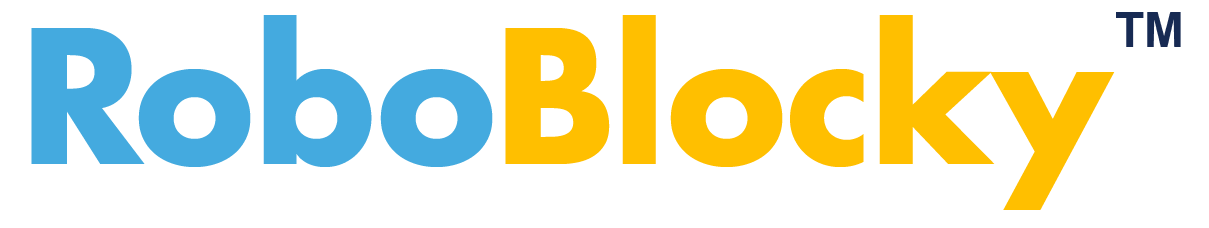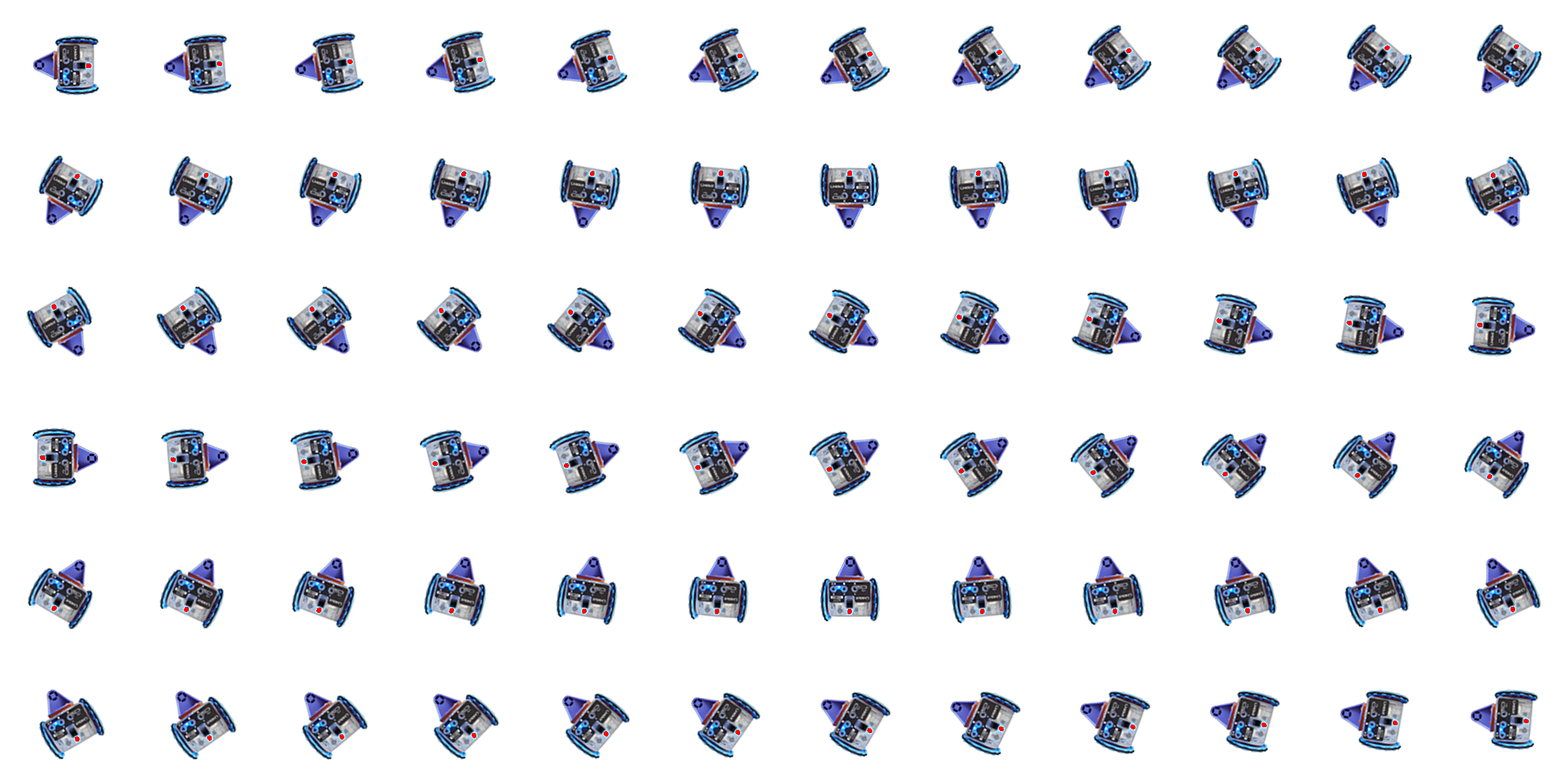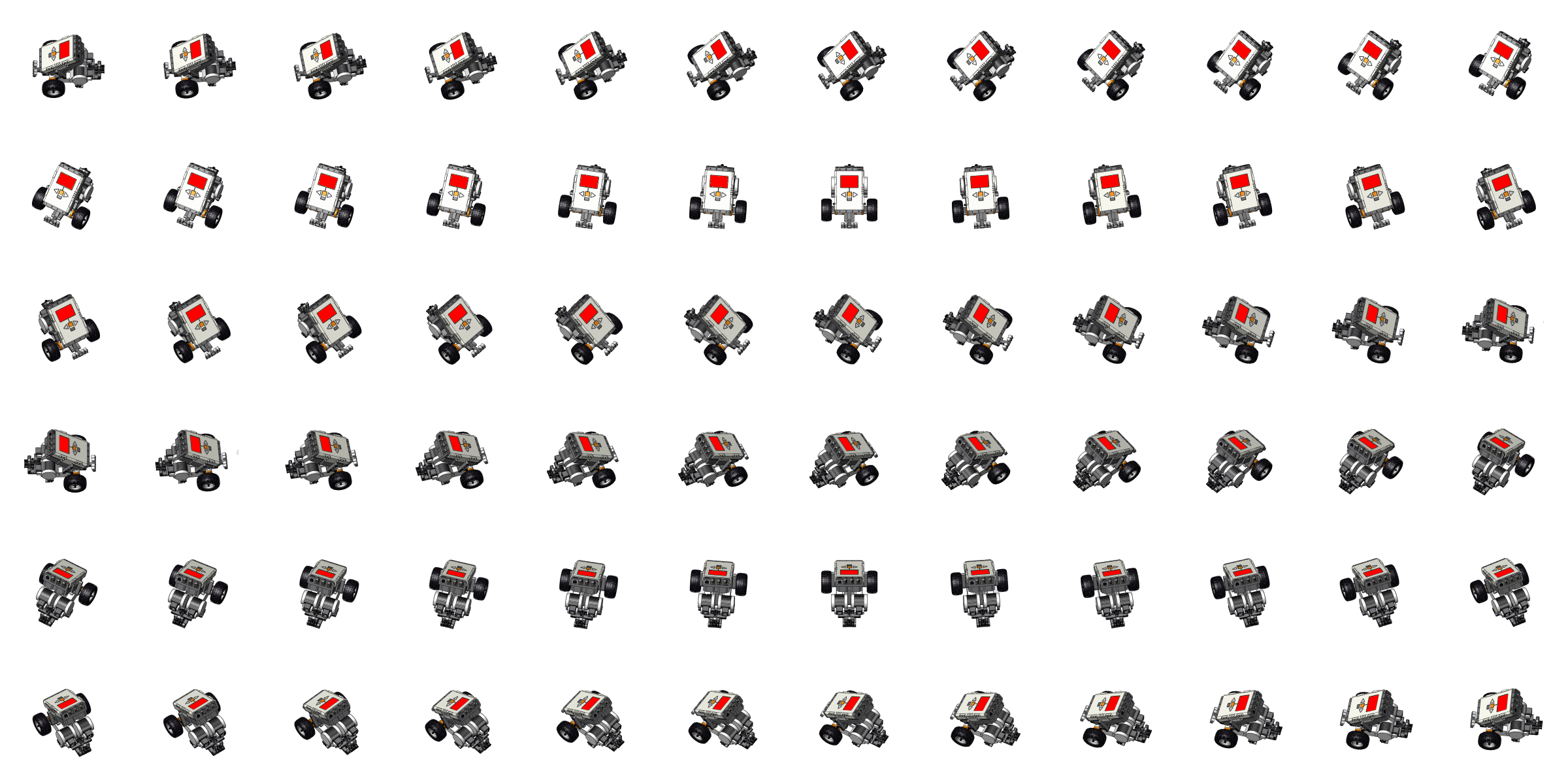Learning Math with Coding and RoboticsGrid Size: S M L Simple View: MinView:
Graphing Linear Equations: Parallel and Perpendicular Lines
 The pre-placed blocks plot a point on the grid and graph a linear equation. Add blocks to draw two more lines, one parallel and one perpendicular to the pre-drawn line, that contain the pre-drawn point on the grid. Each line should be drawn using a different robot.
 Piano Symbol Word APCSP Hardware LArduino Workspace Load Save Ch Ch Shell Console
Lock
roboblockyA271.chTime